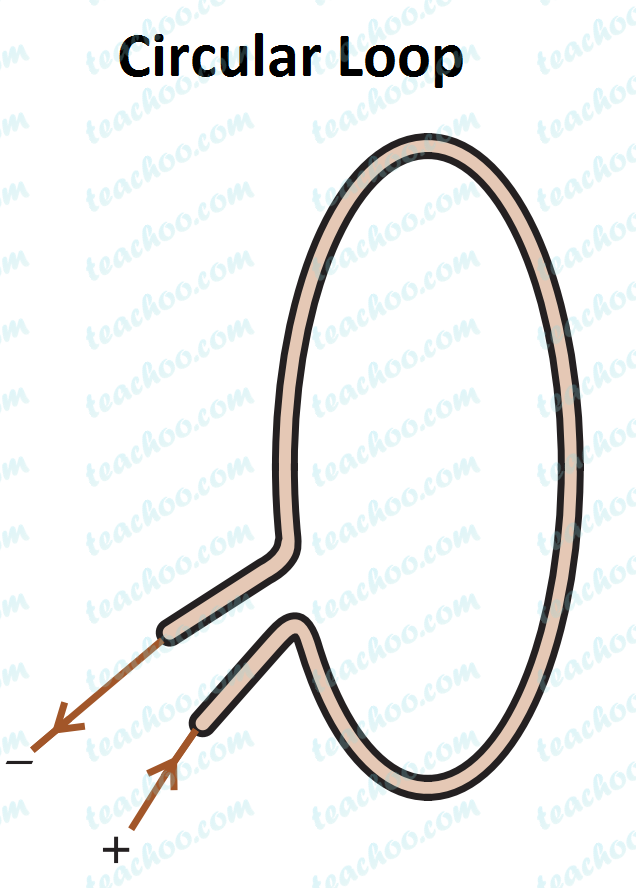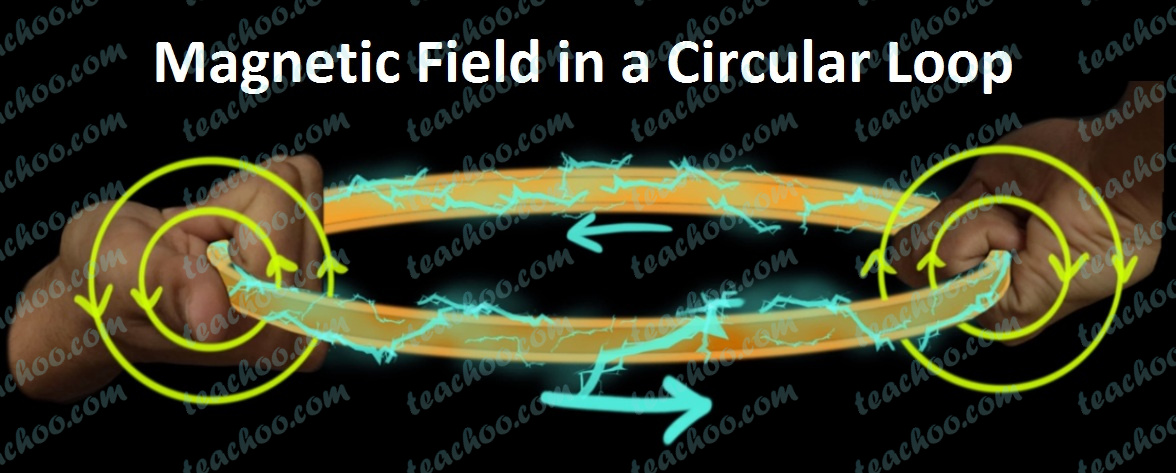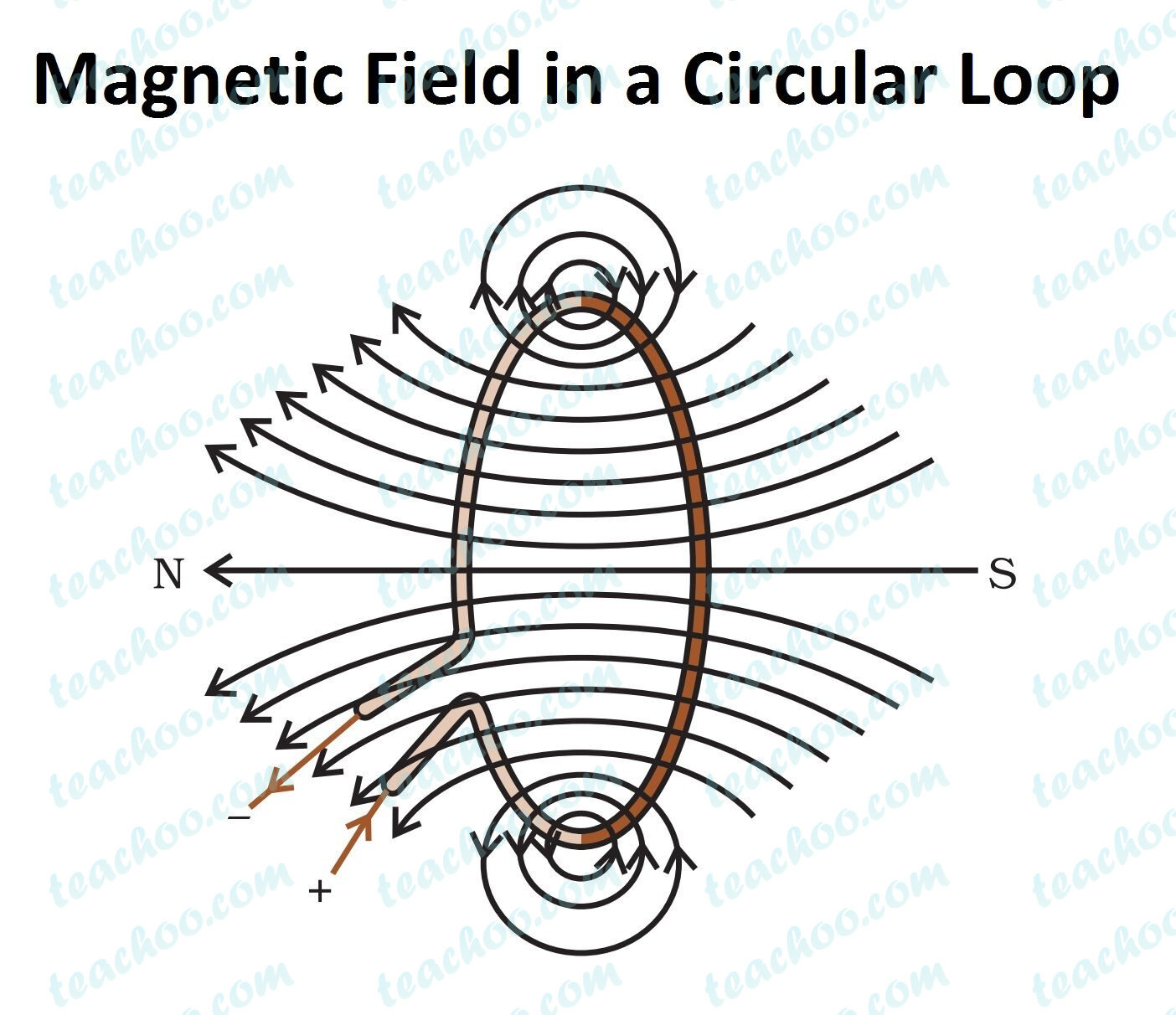Concepts

Class 10
Chapter 13 Class 10 - Magnetic Effects of Electric Current

Let's find Magnetic Field in a Circular LoopWhen we pass electric current through the loop, magnetic field is produced

The direction of magnetic field is given by Right hand thumb Rule

Applying Right hand thumb rule, we get magnetic field asIt is in form of concentric circles near the current carrying loop (wire)As we move away from wires, the circles become bigger and bigger

By the time we reach center of circular loop, the arcs appear as a straight line

## Magnetic field due to current in a circular loop depends on

It depends on

• Magnitude of Electrical Current - More current, more magnetic field
• Radius of circular loop - More radius, less the electric field

Note :

Here we have 1 loop, so there is magnetic field.

If there are 2 loops, magnetic field would be doubled

Similarly, if there are n loops, magnetic field would be n times

Which is the same principle we use in a Solenoid

This is because the current in each circular loop has the same direction
Hence, the field produced will be same in magnitude in direction.
Therefore, the field in each turn adds up.
Hence, if there are n turns, there will be n current carrying loops, and the field will be n times.

## Questions

Q1 Page 229 - Consider a circular loop of wire lying in the plane of the table. Let the current pass through the loop clockwise. Apply the right-hand rule to find out the direction of the magnetic field inside and outside the loop.

Get live Maths 1-on-1 Classs - Class 6 to 12